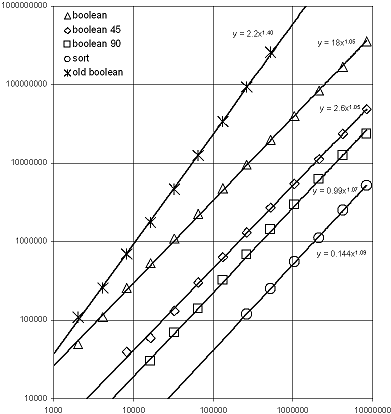# Polygon Set Algorithms Analysis

Most non-trivial algorithms in the Boost.Polygon library are instantiations of generic sweep-line algorithms that provide the capability to perform Manhattan and 45-degree line segment intersection, n-layer map overlay, connectivity graph extraction and clipping/Booleans.  These algorithms have O(n log n) runtime complexity for n equal to input vertices plus intersection vertices.  The arbitrary angle line segment intersection algorithm is not implemented as a sweep-line due to complications related to achieving numerical robustness.  The general line segment intersection algorithm is implemented as an recursive adaptive heuristic divide and conquer in the y dimension followed by sorting line segments in each subdivision by x coordinates and scanning left to right.  By one-dimensional decomposition of the problem space in both x and y the algorithm approximates the optimal O(n log n) Bentley-Ottmann line segment intersection runtime complexity in the common case.  Specific examples of inputs that defeat one dimensional decomposition of the problem space can result in pathological quadratic runtime complexity to which the Bentley-Ottmann algorithm is immune.

Below is shown a log-log plot of runtime versus input size for inputs that increase quadratically in size.  The inputs were generated by pseudo-randomly distributing small axis-parallel rectangles within a square area proportional the the number of rectangles specified for each trial.  In this way the probability of intersections being produced remains constant as the input size grows.  Because intersection vertices are expected to be a constant factor of input vertices we can examine runtime complexity in terms of input vertices.  The operation performed was a union (Boolean OR) of the input rectangles by each of the Manhattan, 45-degree and arbitrary angle Booleans algorithms, which are labeled "boolean 90", "boolean 45" and "boolean".  Also shown in the plot is the performance of the arbitrary angle Booleans algorithm as prior to the addition of divide and conquer recursive subdivision, which was described in the paper presented at boostcon 2009.  Finally, the time required to sort the input points is shown as a common reference for O(n log n) runtime to put the data into context.We can see in the log-log plot that sorting and the three Booleans algorithms provided by the Boost.Polygon library have nearly 45 degree "linear" scaling with empirical exponents just slightly larger than 1.0 and can be observed to scale proportional to O(n log n) for these inputs.  The "old boolean" algorithm presented at boostcon 2009 exhibits scaling close to the expected O(n1.5) scaling.  Because the speedup provided by the divide and conquer approach is algorithmic, the 10X potential performance improvement alluded to in the paper is realized at inputs of 200,000 rectangles and larger.  Even for small inputs of 2K rectangles the algorithm is 2X faster and now can be expected to be roughly as fast as GPC at small scales, while algorithmically faster at large scales.

From the plot we can compare the constant factor performance of the various Booleans algorithms with the runtime of std::sort as a baseline for O(n log n) algorithms.  If you consider sort to be one unit of O(n log n) algorithmic work we can see that Manhattan Booleans cost roughly five units of O(n log n) work, 45-degree  Booleans cost roughly ten units of O(n log n) work and arbitrary angle Booleans cost roughly seventy units of O(n log n) work.  Sorting the input vertices is the first step in a Booleans algorithm and therefore provides a hard lower bound for the runtime of an optimal Booleans algorithm.

One final thing to note about performance of the arbitrary angle Booleans algorithm is that the use of GMP infinite precision rational data type for numerically robust computations can be employed by including boost/polygon/gmp_override.hpp and linking to lgmpxx and lgmp.  This provides 100% assurance that the algorithm will succeed and produce an output snapped to the integer grid with a minimum of one integer grid of error on polygon boundaries upon which intersection points are introduced.  However, the infinite precision data type is never used for predicates (see the boostcon presentation or paper for description of robust predicates) and is only used for constructions of intersection coordinate values in the very rare case that long double computation of the intersection of two line segments fails to produce an intersection point within one integer unit of both line segments.  This means that there is effectively no runtime penalty for the use of infinite precision to ensure 100% robustness.  Most inputs will process through the algorithm without ever resorting to GMP.